• iir数字滤波器设计及软件实现
千次阅读 多人点赞
2021-01-21 12:58:43

# 一、实验目的

• 熟悉用双线性变换法设计 IIR 数字滤波器的原理与方法
• 学会调用 MATLAB 信号处理工具箱中滤波器设计函数（或滤波器设计分析工具fdatool）设计各种 IIR 数字滤波器， 学会根据滤波需求确定滤波器指标参数。
• 掌握 IIR 数字滤波器的 MATLAB 实现方法
• 通过观察滤波器输入输出信号的时域波形及其频谱，建立数字滤波的概念

# 二、实验原理

设计IIR数字滤波器一般采用间接法（脉冲响应不变法和双线性变换法），应用最广泛的是双线性变换法。

基本设计过程如下：

• 先将给定的数字滤波器的指标转换成过渡模拟滤波器的指标
• 设计过渡模拟滤波器
• 将过渡模拟滤波器的系统函数转换成数字滤波器的系统函数，MATLAB信号处理工具箱中的各种 IIR 数字滤波器设计函数都是采用双线
性变换法。

教材第 6 章介绍的滤波器设计函数butter、 cheby1 、cheby2 和 eclip 可以分别被调用来直接设计巴特沃斯、 切比雪夫1、 切比雪夫 2 以及椭圆模拟和数字滤波器。 本实验要求读者调用如上函数直接设计 IIR 数字滤波器。本实验的数字滤波器的 MATLAB 实现是指调用 MATLAB 信号处理工具箱函数 filter 对给定的输入信号 x(n) 进行滤波，得到滤波后的输出信号y(n)。

原理框图如下：matlab
更多相关内容
• 4实验四：IIR数字滤波器设计及软件实现 15 4.1 简述实验目的原理 15 4.2 画出实验主程序框图，打印程序清单 16 4.3：绘制三个分离滤波器的损耗函数曲线 19 4.4：绘制经滤波分离出的三路抑制载波调幅信号的时域波形...
• 数字信号处理实验，包含代码跟实验截图，注释清晰明了，实验结果正确matlab
• MATLAB信号处理工具箱中的各种IIR数字滤波器设计函数都是采用双线性变换法。第六章介绍的滤波器设计函数butter、cheby1 、cheby2 和ellip可以分别被调用来直接设计巴特沃斯、切比雪夫1、切比雪夫2和椭圆模拟和数字...
• 数字信号处理第四次试验：IIR数字滤波器设计及软件实现前言一、实验目的二、实验原理与方法三、实验环境四、实验内容步骤五、实验结果截图（含分析）六、思考题 前言 为了帮助同学们完成痛苦的实验课程设计，本...

# 前言

为了帮助同学们完成痛苦的实验课程设计，本作者将其作出的实验结果及代码贴至CSDN中，供同学们学习参考。如有不足或描述不完善之处，敬请各位指出，欢迎各位的斧正！

# 一、实验目的

（1）熟悉用双线性变换法设计IIR数字滤波器的原理与方法；
（2）学会调用MATLAB信号处理工具箱中滤波器设计函数（或滤波器设计分析工具fdatool）设计各种IIR数字滤波器，学会根据滤波需求确定滤波器指标参数。
（3）掌握IIR数字滤波器的MATLAB实现方法。
（4）通过观察滤波器输入输出信号的时域波形及其频谱，建立数字滤波的概念。

# 二、实验原理与方法

设计IIR数字滤波器一般采用间接法（脉冲响应不变法和双线性变换法），应用最广泛的是双线性变换法。基本设计过程是：①先将给定的数字滤波器的指标转换成过渡模拟滤波器的指标；②设计过渡模拟滤波器；③将过渡模拟滤波器系统函数转换成数字滤波器的系统函数。MATLAB信号处理工具箱中的各种IIR数字滤波器设计函数都是采用双线性变换法。第六章介绍的滤波器设计函数butter、cheby1、cheby2和ellip可以分别被调用来直接设计巴特沃斯、切比雪夫1、切比雪夫2和椭圆模拟和数字滤波器。本实验要求读者调用如上函数直接设计IIR数字滤波器。
本实验的数字滤波器的MATLAB实现是指调用MATLAB信号处理工具箱函数filter对给定的输入信号x(n)进行滤波，得到滤波后的输出信号y(n）。

# 三、实验环境

Matlab 7.0及Matlab 2018b

# 四、实验内容及步骤

（1）调用信号产生函数mstg产生由三路抑制载波调幅信号相加构成的复合信号st，该函数还会自动绘图显示st的时域波形和幅频特性曲线，如图所示。由图可见，三路信号时域混叠无法在时域分离。但频域是分离的，所以可以通过滤波的方法在频域分离，这就是本实验的目的。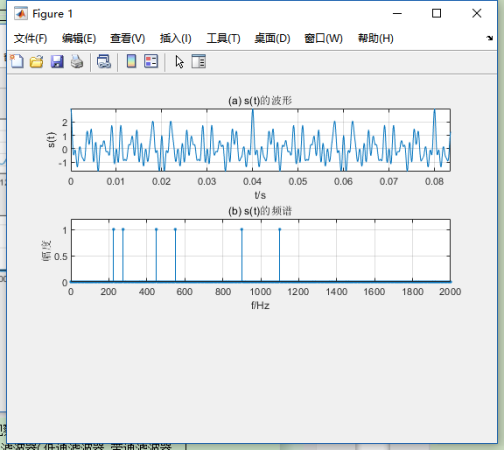（2）要求将st中三路调幅信号分离，通过观察st的幅频特性曲线，分别确定可以分离st中三路抑制载波单频调幅信号的三个滤波器（低通滤波器、带通滤波器、高通滤波器）的通带截止频率和阻带截止频率。要求滤波器的通带最大衰减为0.1dB,阻带最小衰减为60dB。
提示：抑制载波单频调幅信号的数学表示式为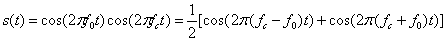其中， c o s ( 2 π f c t ) cos(2\pi f_ct) 称为载波， f c f_c 为载波频率， c o s ( 2 π f 0 t ) cos(2\pi f_0t) 称为单频调制信号， f 0 f_0 为调制正弦波信号频率，且满足 f c > f 0 f_c>f_0 。由上式可见，所谓抑制载波单频调幅信号，就是2个正弦信号相乘，它有2个频率成分：和频 f c + f 0 f_c+f_0 和差频 f c − f 0 f_c-f_0 ，这2个频率成分关于载波频率 f c f_c 对称。所以，1路抑制载波单频调幅信号的频谱图是关于载波频率 f c f_c 对称的2根谱线，其中没有载频成分，故取名为抑制载波单频调幅信号。容易看出，图中三路调幅信号的载波频率分别为250Hz、500Hz、1000Hz。如果调制信号m(t)具有带限连续频谱，无直流成分，则 s ( t ) = m ( t ) c o s ( 2 π f c t ) s(t)=m(t)cos(2\pi f_ct) 就是一般的抑制载波调幅信号。其频谱图是关于载波频率 f c f_c 对称的2个边带（上下边带），在专业课通信原理中称为双边带抑制载波(DSB-SC)调幅信号,简称双边带(DSB)信号。如果调制信号m(t)有直流成分，则 s ( t ) = m ( t ) c o s ( 2 π f c t ) s(t)=m(t)cos(2\pi f_ct) 就是一般的双边带调幅信号。其频谱图是关于载波频率 f c f_c 对称的2个边带（上下边带），并包含载频成分。
（3）编程序调用MATLAB滤波器设计函数ellipord和ellip分别设计这三个椭圆滤波器，并绘图显示其幅频响应特性曲线。
（4）调用滤波器实现函数filter，用三个滤波器分别对信号产生函数mstg产生的信号st进行滤波，分离出st中的三路不同载波频率的调幅信号 y 1 ( n ) y_1(n) y 2 ( n ) y_2(n) y 3 ( n ) y_3(n) ，并绘图显示 y 1 ( n ) y_1(n) y 2 ( n ) y_2(n) y 3 ( n ) y_3(n) 的时域波形，观察分离效果。

# 五、实验结果截图（含分析）

滤波器参数及实验程序清单
1、滤波器参数选取
观察图可知，三路调幅信号的载波频率分别为250Hz、500Hz、1000Hz。带宽（也可以由信号产生函数mstg清单看出）分别为50Hz、100Hz、200Hz。所以，分离混合信号st中三路抑制载波单频调幅信号的三个滤波器（低通滤波器、带通滤波器、高通滤波器）的指标参数选取如下：
对载波频率为250Hz的条幅信号，可以用低通滤波器分离，其指标为
带截止频率 f p f_p =280Hz，通带最大衰减 a p a_p =0.1dB；
阻带截止频率 f s f_s =450Hz，阻带最小衰减 a s a_s =60dB，
对载波频率为500Hz的条幅信号，可以用带通滤波器分离，其指标为
带截止频率 f p i f_{pi} =440Hz， f p u f_{pu} =560Hz，通带最大衰减 a p a_p =0.1dB；
阻带截止频率 f s i f_{si} =275Hz， f s u f_{su} =900Hz，阻带最小衰减 a s a_s =60dB，
对载波频率为1000Hz的条幅信号，可以用高通滤波器分离，其指标为
带截止频率 f p f_p =890Hz，通带最大衰减 a p a_p =0.1dB；
阻带截止频率 f s f_s =550Hz，阻带最小衰减 a s a_s =60dB，
说明：（1）为了使滤波器阶数尽可能低，每个滤波器的边界频率选择原则是尽量使滤波器过渡带宽尽可能宽。
（2）与信号产生函数mstg相同，采样频率Fs=10kHz。
（3）为了滤波器阶数最低，选用椭圆滤波器。
按照图4.2所示的程序框图编写的实验程序为exp4.m。

实验程序清单

%IIR数字滤波器设计及软件实现
clear;close all
Fs=10000;T=1/Fs;%采样频率
%调用信号产生函数mstg产生由三路抑制载波调幅信号相加构成的复合信号st
st=mstg;
%低通滤波器设计与实现=========================================
fp=280;fs=450;
wp=2*fp/Fs;ws=2*fs/Fs;rp=0.1;rs=60;%OF指标(低通滤波器的通、阻带边界频)
[N,wp]=ellipord(wp,ws,rp,rs);%调用ellipord计算椭圆DF阶数N和通带截止频率wp
[B,A]=ellip(N,rp,rs,wp);%调用ellip计算椭圆带通DF系统函数系数向量B和A
y1t=filter(B,A,st);%滤波器软件实现
%低通滤波器设计与实现绘图部分
figure(2);subplot(3,1,1);
myplot(B,A);%调用绘图函数myplot绘制损耗函数曲线
yt='y_1(t)';
subplot(3,1,2);tplot(y1t,T,yt);%调用绘图函数tplot绘制滤波器输出波形
%带通滤波器设计与实现=================================================
fpl=440;fpu=560;fsl=275;fsu=900;
wp=[2*fpl/Fs,2*fpu/Fs];ws=[2*fsl/Fs,2*fsu/Fs];rp=0.1;rs=60;
[N,wp]=ellipord(wp,ws,rp,rs);%调用e1lipord计算椭圆DF阶数N和通带截止频率wp
[B,A]=ellip(N,rp,rs,wp);%调用ellip计算椭圆带通DF系统函数系数向量B和A
y2t=filter(B,A,st);%滤波器软件实现
%带通滤波器设计与实现绘图部分
figure(2);subplot(3,1,1);
myplot(B,A);%调用绘图函数myplot绘制损耗函数曲线
yt='y_2(t)';
subplot(3,1,2);tplot(y2t,T,yt);axis([0,0.08,-1.2,1.2])%调用绘图函数tplot绘制滤波器输出波形
%高通滤波器设计与实现================================================
fp=890;fs=600;
wp=2*fp/Fs;ws=2*fs/Fs;rp=0.1;rs=60;%OF指标(低通滤波器的通、阻带边界频)
[N,wp]=ellipord(wp,ws,rp,rs);%调用ellipord计算椭圆DF阶数N和通带截止频率wp
[B,A]=ellip(N,rp,rs,wp,'high');%调用ellip计算椭圆带通DF系统函数系数向量B和A
y3t=filter(B,A,st);%滤波器软件实现
%高低通滤波器设计与实现绘图部分
figure(2);subplot(3,1,1);
myplot(B,A);%调用绘图函数myplot绘制损耗函数曲线
yt='y_3(t)';
subplot(3,1,2);tplot(y3t,T,yt);axis([0,0.08,-1.2,1.2])

function tplot(xn,T,yn)
n=0:length(xn)-1;
t=n*T;
plot(t,xn);
xlabel('t/s');
ylabel('yn');
axis([0,t(end),min(xn),1.2*max(xn)]);

function myplot(B,A)
[H,W]=freqz(B,A,1000);
m=abs(H);
plot(W/pi,20*log10(m/max(m)));
grid on;
xlabel('\omega/\pi');
ylabel('幅度(dB)')
axis([0,1,-80,5]);
title('损耗函数曲线');

function st=mstg
%产生信号序列向量st,并显示st的时域波形和频谱
%st=mstg返回三路调幅信号相加形成的混合信号，长度N=1600
N=1600%N为信号st的长度。
Fs=10000;T=1/Fs;Tp=N*T;%采样频率Fs=10kHz，Tp为采样时间
t=0:T:(N-1)*T;k=0:N-1;f=k/Tp;
fc1=Fs/10;%第1路调幅信号的载波频率fc1=1000Hz,
fm1=fc1/10;%第1路调幅信号的调制信号频率fm1=100Hz
fc2=Fs/20;%第2路调幅信号的载波频率fc2=500Hz
fm2=fc2/10;%第2路调幅信号的调制信号频率fm2=50Hz
fc3=Fs/40;%第3路调幅信号的载波频率fc3=250Hz,
fm3=fc3/10;%第3路调幅信号的调制信号频率fm3=25Hz
xt1=cos(2*pi*fm1*t).*cos(2*pi*fc1*t);%产生第1路调幅信号
xt2=cos(2*pi*fm2*t).*cos(2*pi*fc2*t);%产生第2路调幅信号
xt3=cos(2*pi*fm3*t).*cos(2*pi*fc3*t);%产生第3路调幅信号
st=xt1+xt2+xt3;%三路调幅信号相加
fxt=fft(st,N);%计算信号st的频谱
subplot(3,1,1)
plot(t,st);grid;xlabel('t/s');ylabel('s(t)');
axis([0,Tp/8,min(st),max(st)]);title('(a)s(t)的波形')
subplot(3,1,2)
stem(f,abs(fxt)/max(abs(fxt)),'.');grid;title('(b)s(t)的频谱')
axis([0,Fs/5,0,1.2]);
xlabel('f/Hz');ylabel('幅度')


实验程序运行结果及分析讨论
实验4程序运行结果如图所示。由图可见，三个分离滤波器指标参数选取正确，算耗函数曲线达到所给指标。分离出的三路信号y1(n)，y2(n)和y3(n)的波形是抑制载波的单频调幅波。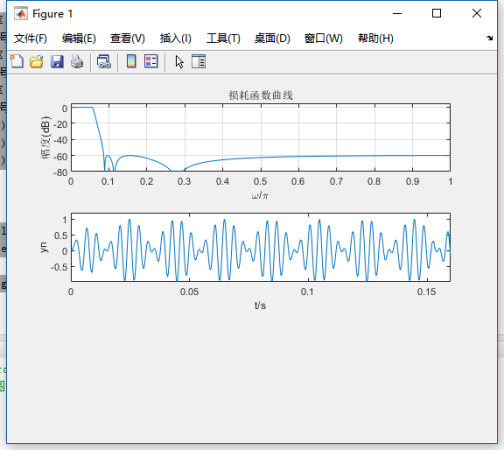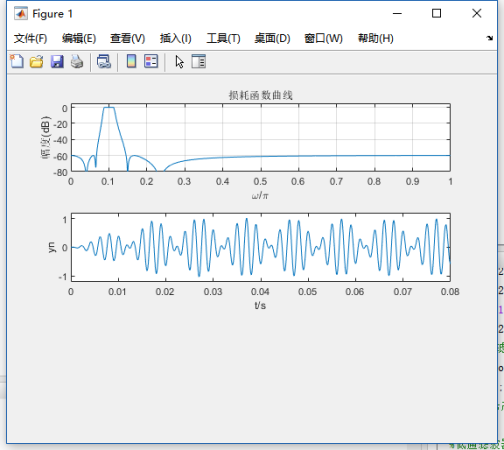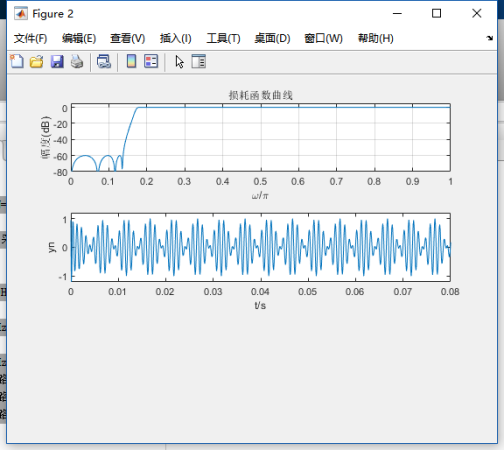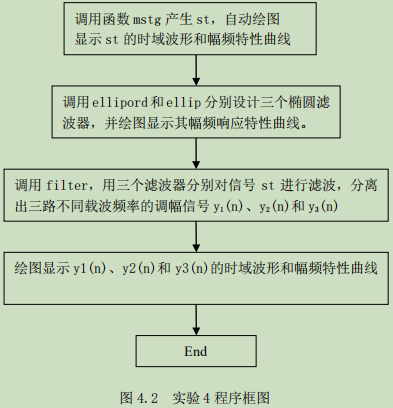# 六、思考题

（1）请阅读信号产生函数mstg，确定三路调幅信号的载波频率和调制信号频率。
（2）信号产生函数mstg中采样点数N=800，对st进行N点FFT可以得到6根理想谱线。如果取N=1000，可否得到6根理想谱线？为什么？N=2000呢？请改变函数mstg中采样点数N的值，观察频谱图验证您的判断是否正确。
（3）修改信号产生函数mstg，给每路调幅信号加入载波成分，产生调幅（AM）信号，重复本实验，观察AM信号与抑制载波调幅信号的时域波形及其频谱的差别。
提示：AM信号表示式： s ( t ) = [ 1 + c o s ( 2 π f 0 t ) ] c o s ( 2 π f c t ) s(t)=[1+cos(2\pi f_0t)]cos(2\pi f_ct)
答：（1）观察图可知，三路调幅信号的载波频率分别为250Hz、500Hz、1000Hz。带宽（也可以由信号产生函数mstg清单看出）分别为50Hz、100Hz、200Hz。所以，分离混合信号st中三路抑制载波单频调幅信号的三个滤波器（低通滤波器、带通滤波器、高通滤波器）的指标参数选取如下：
对载波频率为250Hz的条幅信号，可以用低通滤波器分离，其指标为
带截止频率 f p f_p =280Hz，通带最大衰减 a p a_p =0.1dB；
阻带截止频率 f s f_s =450Hz，阻带最小衰减 a s a_s =60dB，
对载波频率为500Hz的条幅信号，可以用带通滤波器分离，其指标为
带截止频率 f p i f_{pi} =440Hz， f p u f_{pu} =560Hz，通带最大衰减 a p a_p =0.1dB；
阻带截止频率 f s i f_{si} =275Hz， f s u f_{su} =900Hz，阻带最小衰减 a s a_s =60dB，
对载波频率为1000Hz的条幅信号，可以用高通滤波器分离，其指标为
带截止频率 f p f_p =890Hz，通带最大衰减 a p a_p =0.1dB；
阻带截止频率 f s f_s =550Hz，阻带最小衰减 a s a_s =60dB，
（3）因为信号st是周期序列，谱分析时要求观察时间为整数倍周期。所以，
本题的一般解答方法是，先确定信号st的周期，在判断所给采样点数N对应的观察时间Tp=NT是否为st的整数个周期。但信号产生函数mstg产生的信号st共有6个频率成分，求其周期比较麻烦，故采用下面的方法解答。
分析发现，st的每个频率成分都是25Hz的整数倍。采样频率Fs=10kHz=25×400Hz，即在25Hz的正弦波的1个周期中采样400点。所以，当N为400的整数倍时一定为st的整数个周期。因此，采样点数N=800和N=2000时，对st进行N点FFT可以得到6根理想谱线。如果取N=1000，不是400的整数倍，不能得到6根理想谱线。

展开全文matlab
• 数字信号处理：IIR数字滤波器设计及软件实现 1．实验原理 设计IIR数字滤波器一般采用间接法（脉冲响应不变法和双线性变换法），应用最广泛的是双线性变换法。基本设计过程是：①先将给定的数字滤波器的指标转换成...

# 数字信号处理：IIR数字滤波器设计及软件实现

### 1．实验原理

设计IIR数字滤波器一般采用间接法（脉冲响应不变法和双线性变换法），应用最广泛的是双线性变换法。基本设计过程是：①先将给定的数字滤波器的指标转换成过渡模拟滤波器的指标； ②设计过渡模拟滤波器；③将过渡模拟滤波器系统函数转换成数字滤波器的系统函数。MATLAB信号处理工具箱中的各种IIR数字滤波器设计函数都是采用双线性变换法。第六章介绍的滤波器设计函数butter、cheby1 、cheby2 和ellip可以分别被调用来直接设计巴特沃斯、切比雪夫1、切比雪夫2和椭圆模拟和数字滤波器。本实验要求读者调用如上函数直接设计IIR数字滤波器。
本实验的数字滤波器的MATLAB实现是指调用MATLAB信号处理工具箱函数filter对给定的输入信号x(n)进行滤波，得到滤波后的输出信号y(n）。

### 2. 实验内容及步骤

（1）调用信号产生函数mstg产生由三路抑制载波调幅信号相加构成的复合信号st，该函数还会自动绘图显示st的时域波形和幅频特性曲线，如图10.4.1所示。由图可见，三路信号时域混叠无法在时域分离。但频域是分离的，所以可以通过滤波的方法在频域分离，这就是本实验的目的。

（2）要求将st中三路调幅信号分离，通过观察st的幅频特性曲线，分别确定可以分离st中三路抑制载波单频调幅信号的三个滤波器（低通滤波器、带通滤波器、高通滤波器）的通带截止频率和阻带截止频率。要求滤波器的通带最大衰减为0.1dB,阻带最小衰减为60dB。
提示：抑制载波单频调幅信号的数学表示式为

其中， 称为载波，fc为载波频率， 称为单频调制信号，f0为调制正弦波信号频率，且满足 。由上式可见，所谓抑制载波单频调幅信号，就是2个正弦信号相乘，它有2个频率成分：和频 和差频 ，这2个频率成分关于载波频率fc对称。所以，1路抑制载波单频调幅信号的频谱图是关于载波频率fc对称的2根谱线，其中没有载频成分，故取名为抑制载波单频调幅信号。容易看出，图10.4.1中三路调幅信号的载波频率分别为250Hz、500Hz、1000Hz。如果调制信号m(t)具有带限连续频谱，无直流成分，则 就是一般的抑制载波调幅信号。其频谱图是关于载波频率fc对称的2个边带（上下边带），在专业课通信原理中称为双边带抑制载波 (DSB-SC) 调幅信号,简称双边带 (DSB) 信号。如果调制信号m(t)有直流成分，则 就是一般的双边带调幅信号。其频谱图是关于载波频率fc对称的2个边带（上下边带），并包含载频成分。
（3）编程序调用MATLAB滤波器设计函数ellipord和ellip分别设计这三个椭圆滤波器，并绘图显示其幅频响应特性曲线。
（4）调用滤波器实现函数filter，用三个滤波器分别对信号产生函数mstg产生的信号st进行滤波，分离出st中的三路不同载波频率的调幅信号y1(n)、y2(n)和y3(n)， 并绘图显示y1(n)、y2(n)和y3(n)的时域波形，观察分离效果。

mstg信号生成函数

function st= mstg
%产生信号序列向量st，并显示st的时域波形和频谱
%st=mstg返回三路调幅信号相加形成的混合信号，长度N=1600
N=1600;  %N为信号st的长度
Fs=10000;
T=1/Fs;
Tp=N*T; %采样频率Fs=10kHz，Tp为采样时间
t=0:T:(N-1)*T;
k=0:N-1;
f=k/Tp;
fc1=Fs/10;   %第1路调幅信号的载波频率fcl=1000Hz
fml=fc1/10;  %第1路调幅信号的调制信号频率fml=100Hz
fc2=Fs/20;   %第2路调幅信号的载波频率fc2=500Hz
fm2=fc2/10;  %第2路调幅信号的调制信号频率fm2=50Hz
fc3=Fs/40;  %第3路调幅信号的载波频率fc3=250Hz
fm3=fc3/10;  %第3路调幅信号的调制信号频率fm3=25Hz
xt1=cos(2*pi*fml*t).*cos(2*pi*fc1*t);  %产生第1路调幅信号
xt2=cos(2*pi*fm2*t).*cos(2*pi*fc2*t);  %产生第2路调幅信号
xt3=cos(2*pi*fm3*t).*cos(2*pi*fc3*t);  %产生第3路调幅信号
st=xt1+xt2+xt3;%三路调幅信号相加
fxt=fft(st,N);%计算信号st的频谱
%====以下为绘图部分，绘制st的时域波形和幅频特性曲线===========
subplot(3,1,1)
plot(t,st);
grid;xlabel('t/s');ylabel('s（t）');
axis([0,Tp/8,min(st),max(st)]);
title('（a）s（t）的波形');
subplot(3,1,2);
stem(f,abs(fxt)/max(abs(fxt)),'.');grid;
title('（b）s（t）的频谱');
axis([0,Fs/5,0,1.2]);
xlabel('f/Hz');ylabel('幅度');
end



main主函数：

clear all;close all;
Fs=10000;T=1/Fs;
st=mstg;
%低通滤波器
fp=280;fs=450;
wp=2*fp/Fs;ws=2*fs/Fs;rp=0.1;rs=60;
[N,wpo]=ellipord(wp,ws,rp,rs);  %计算椭圆低通模拟滤波器阶数和通带边界频率
[B,A]=ellip(N,rp,rs,wpo);   %计算低通模拟滤波器系统函数系数
ylt=filter(B,A,st);
[H,w]=freqz(B,A,1000);    %求解离散系统频率响应的函数fregz()
m=abs(H);
loseH=20*log10(m/max(m));
subplot(2,1,1);plot(w/pi,loseH);
xlabel('w/pi');ylabel('幅度/dB');
subplot(2,1,2);plot(ylt);
xlabel('t/s');ylabel('y(t)');
%%
%带通滤波器
fpl=440;fpu=560;fsl=275;fsu=900;
wp=[2*fpl/Fs,2*fpu/Fs];
ws=[2*fsl/Fs,2*fsu/Fs];
rp=0.1;rs=60;
[N,wp]=ellipord(wp,ws,rp,rs);
[B,A]=ellip(N,rp,rs,wp);
y2t=filter(B,A,st);
[H,w]=freqz(B,A,1000);    %求解离散系统频率响应的函数fregz()
m=abs(H);
loseH=20*log10(m/max(m));
subplot(2,1,1);plot(w/pi,loseH);
xlabel('w/pi');ylabel('幅度/dB');
subplot(2,1,2);plot(ylt);
xlabel('t/s');ylabel('y(t)');

%%
%高通滤波器
fp=890;fs=600;
wp=2*fp/Fs;ws=2*fs/Fs;rp=0.1;rs=60;
[N,wp]=ellipord(wp,ws,rp,rs);
[B,A]=ellip(N,rp,rs,wp,'high');
y3t=filter(B,A,st);
[H,w]=freqz(B,A,1000);    %求解离散系统频率响应的函数fregz()
m=abs(H);
loseH=20*log10(m/max(m));
subplot(2,1,1);plot(w/pi,loseH);
xlabel('w/pi');ylabel('幅度/dB');
subplot(2,1,2);plot(ylt);
xlabel('t/s');ylabel('y(t)');


展开全文• 信息与机电工程系专业：年级： 2015级姓名：学号：实验课程：数字信号处理实验室号：_ 金综B706 实验设备号：实验时间： 2015.12.31 指导教师签字：成绩：实验四IIR数字滤波器设计及软件实现一、实验目的(1)熟悉用...

福建农林大学金山学院信息工程类实验报告

系：信息与机电工程系专业：年级： 2015级

姓名：学号：实验课程：数字信号处理

实验室号：_ 金综B706 实验设备号：实验时间： 2015.12.31 指导教师签字：成绩：

实验四IIR数字滤波器设计及软件实现

一、实验目的

(1)熟悉用双线性变换法设计IIR数字滤波器的原理与方法；

(2)学会调用MATLAB信号处理工具箱中滤波器设计函数(或滤波器设

计分析工具fdatool)设计各种IIR数字滤波器，学会根据滤波需求确定滤波器指

标参数。

(3)掌握IIR数字滤波器的MATLAB实现方法。

(3)通过观察滤波器输入输出信号的时域波形及其频谱，建立数字滤波的

概念。

二、实验原理

设计IIR数字滤波器一般采用间接法(脉冲响应不变法和双线性变换法)，

应用最广泛的是双线性变换法。基本设计过程是：①先将给定的数字滤波器的指

标转换成过渡模拟滤波器的指标；②设计过渡模拟滤波器；③将过渡模拟滤波

器系统函数转换成数字滤波器的系统函数。MATLAB信号处理工具箱中的各种IIR数字滤波器设计函数都是采用双线性变换法。第六章介绍的滤波器设计函数butter、cheby1 、cheby2 和ellip可以分别被调用来直接设计巴特沃斯、切比雪

夫1、切比雪夫2和椭圆模拟和数字滤波器。本实验要求读者调用如上函数直接

设计IIR数字滤波器。

本实验的数字滤波器的MATLAB实现是指调用MATLAB信号处理工具箱

函数filter对给定的输入信号x(n)进行滤波，得到滤波后的输出信号y(n)。

三、实验内容及步骤

(1)调用信号产生函数mstg产生由三路抑制载波调幅信号相加构成的复合

信号st，该函数还会自动绘图显示st的时域波形和幅频特性曲线，如图10.4.1

所示。由图可见，三路信号时域混叠无法在时域分离。但频域是分离的，所以可

展开全文• 实验3 IIR数字滤波器设计及软件实现 一、实验安全规则（本实验项目安全注意事项） 1.数字信号处理DSP应用实验在计算机Matlab上用仿真方式完成，不需要硬件设备，请不要开启计算机桌台上的其它硬件设备； 2.实验...matlab
• （2）学会调用MATLAB信号处理工具箱中滤波器设计函数（或滤波器设计分析工具fdatool）设计各种IIR数字滤波器，学会根据滤波需求确定滤波器指标参数。 （3）掌握IIR数字滤波器的MATLAB实现方法。 （3）通过观察...
•文档
• 实验四IIR数字滤波器设计及软件实现实验报告一、实验目的(1)熟悉用双线性变换法设计IIR数字滤波器的原理与方法；(2)学会调用MATLAB信号处理工具箱中滤波器设计函数(或滤波器设计分析工具fdatool)设计各种IIR数字...
•文档
•文档
• 实验4IIR数字滤波器设计及软件实现-实验报告汇总【甄选文档】.pdf
• 通信数字信号处理。 设计数字滤波器 用matlab直接打开
• 实验四IIR 数字滤波器 设计及软件实现.doc实验四 IIR 数字滤波器 设计及软件实现1.实验目的1熟悉用双线性变换法设计IIR数字滤波器的原理与方法;2学会调用MATLAB信号处理工具箱中滤波器设计函数或滤波器设计分析工具...
• 2.学会调用MATLAB信号处理工具箱中滤波器设计函数（或滤波器设计分析工具FDATool）设计各种IIR数字滤波器，学会根据滤波需求确定滤波器指标参数。 3.掌握IIR数字滤波器的MATLAB实现方法。 4.通过观察滤波器输入、...matlab
• 10.4实验四IIR数字滤波器设计及软件实现10.4.1实验指导1．实验目的(1)熟悉用双线性变换法设计IIR数字滤波器的原理与方法；(2)学会调用MATLAB信号处理工具箱中滤波器设计函数(或滤波器设计分析工具fdatool)设计各种...
• iir数字滤波器设计及其matlab实现 1IIR 数字滤波器设计及其 MATLAB 实现自动化学院 杨梅 201422070125摘 要IIR 数 字 滤 波 器 在 设 计 上 可 以 借 助 成 熟 的 模 拟 滤 波 器 的 成 果 ，如 巴 特 沃 斯 、 契 ...
• 　实现数字滤波器的方法有两种，一是采用计算机软件进行，就是把所要完成的工作通过程序让计算机来实现；二是设计专用的数字处理硬件。这个地方主要用到的就是第一种方法。即是用Mafiab提供的信号处理工具箱来实现...
• IIR数字滤波器是数字信号处理的重要工具之一。利用Matlab信号处理工具箱和使用切比雪夫法设计IIR数字滤波器,并进行仿真。仿真结果表明,设计过程简单方便。
• 低通滤波器设计：1、基本指标低通滤波器顾名思义就是低频率成分通过，高频率成分截止，那么在设计一个低通滤波器时首先要明白想要截止多大的频率。比如想截掉4Hz以上的信号，理想状态下就是将4Hz以上的信号成分...
• 　实现数字滤波器的方法有两种，一是采用计算机软件进行，就是把所要完成的工作通过程序让计算机来实现；二是设计专用的数字处理硬件。这个地方主要用到的就是种方法。即是用Mafiab提供的信号处理工具箱来实现数字...
• 东南大学09级数字信号处理的实验 实验四报告 电子版 IIR数字滤波器
•文档...